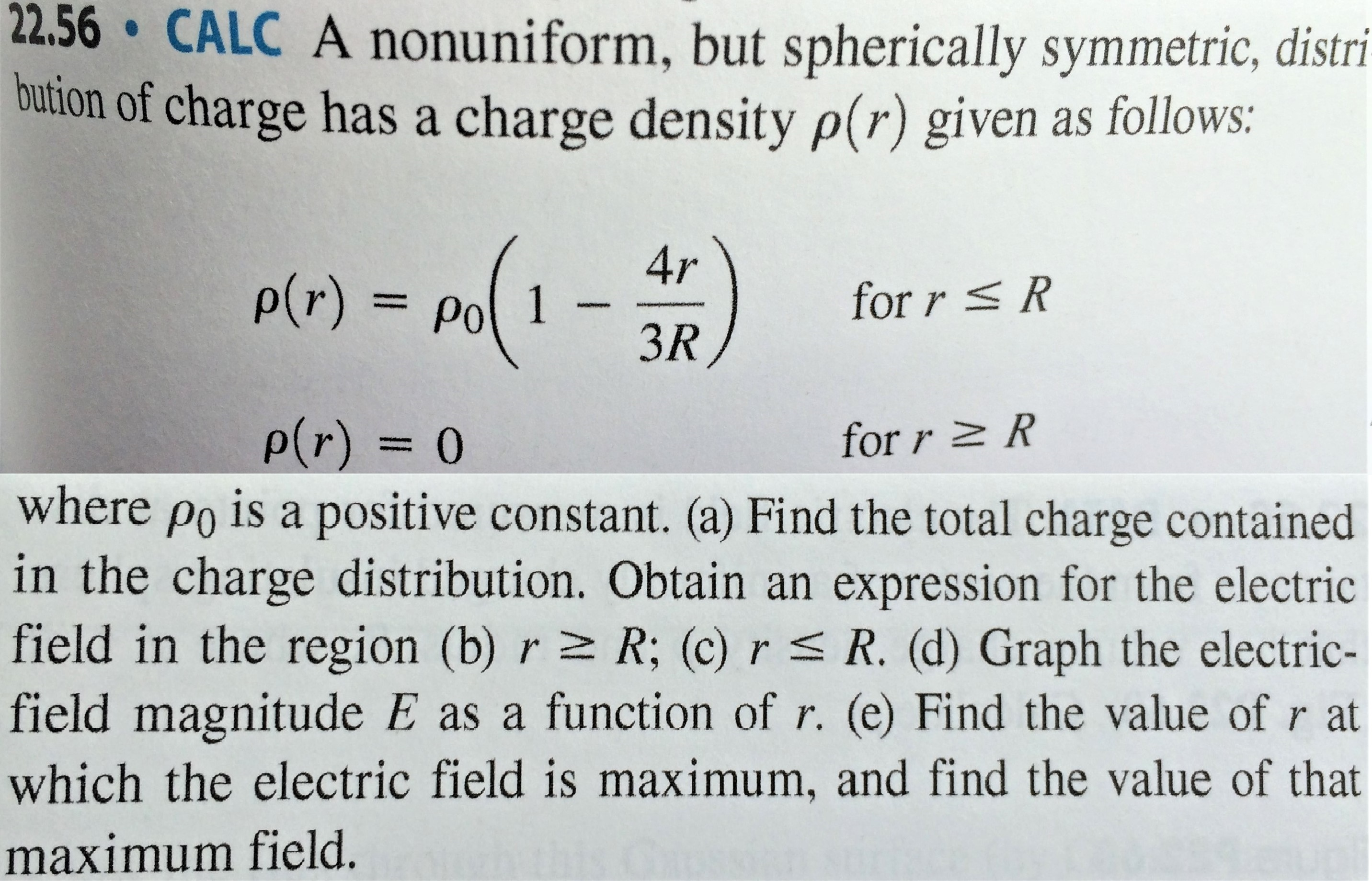# 22.53 CALC A nonuniform, but spherically symmetric, dis-tribution of charge has a charge density p(r) given as follows:Ho-(1-2rp(r)= Pofor r RRfor r RP(r) 0where po 30/TR is a positive constant. (a) Show that the totalcharge contained in the charge distribution is Q. (b) Show that theelectric field in the region r 2 R is identical to that produced by apoint charge Q at r = 0. (c) Obtain an expression for the electricfield in the region r = R. (d) Graph the electric-field magnitude E(e) Find the value of r at which the electric fieldis maximum, and find the value of that maximum field.as a function of r.slab of insulatingnar-22.5 22.56 CALC A nonuniform, but spherically symmetric, distri-bution of charge has a charge density p(r) given as follows:4rP(r) = Afor r R3R,P(r)= 0for r Rwhere po is a positive constant. (a) Find the total charge containedin the charge distribution. Obtain an expression for the electricfield in the region (b) r z R; (c) rs R. (d) Graph the electric-field magnitude E as a function of r. (e) Find the value of r atwhich the electric field is maximum, and find the value of thatmaximum field.

Question
51 views

(Problems in images below.)

Both problems require a volume charge integration of the given charge density functions. Technically, a volume integration is a triple integral. However, since the charge density is spherically symmetric, this reduces to a one-dimensional integration.help_outlineImage Transcriptionclose22.53 CALC A nonuniform, but spherically symmetric, dis- tribution of charge has a charge density p(r) given as follows: Ho-(1-2 r p(r)= Po for r R R for r R P(r) 0 where po 30/TR is a positive constant. (a) Show that the total charge contained in the charge distribution is Q. (b) Show that the electric field in the region r 2 R is identical to that produced by a point charge Q at r = 0. (c) Obtain an expression for the electric field in the region r = R. (d) Graph the electric-field magnitude E (e) Find the value of r at which the electric field is maximum, and find the value of that maximum field. as a function of r. slab of insulating nar- 22.5 fullscreenhelp_outlineImage Transcriptionclose22.56 CALC A nonuniform, but spherically symmetric, distri- bution of charge has a charge density p(r) given as follows: 4r P(r) = A for r R 3R, P(r)= 0 for r R where po is a positive constant. (a) Find the total charge contained in the charge distribution. Obtain an expression for the electric field in the region (b) r z R; (c) rs R. (d) Graph the electric- field magnitude E as a function of r. (e) Find the value of r at which the electric field is maximum, and find the value of that maximum field. fullscreen
check_circle

Step 1

a) Total charge is,

Step 2

b) Using Gauss law,

Step 3

c) Total char...

### Want to see the full answer?

See Solution

#### Want to see this answer and more?

Solutions are written by subject experts who are available 24/7. Questions are typically answered within 1 hour.*

See Solution
*Response times may vary by subject and question.
Tagged in

### Electric Charges and Fields